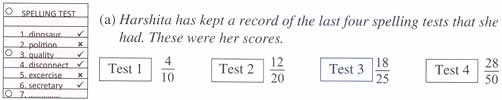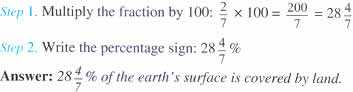Tuesday , July 5 2022# NCERT 5th Class (CBSE) Mathematics: Percentage

## FRACTION AND PER CENTS

### Converting Fractions to Per Cents

A. Fractions that have equivalent fractions with denominator 100In which test did she do the best?

Since all the tests were based on different number of marks, we cannot compare them. Instead we could find the percentage she got in each test in order to be able to compare.

To express each fraction as a percentage:

Step 1. Write the equivalent fraction with a denominator of 100.

Step 2. Write the answer with a percentage sign (%).

Test 1: 4/10 = 4 × 10/10 × 10 = 40/100 = 40%

Test 2: 12/20 = 12 × 5/20 × 5 = 60/100 = 60%

Test 3: 18/25 = 18 × 4/25 × 4 = 72/100 = 72%

Test 4: 28/50 = 28 × 2/50 × 2 = 56/100 = 56%

Now since all the figures are in per cents we can see that Harshita did the best in Test 3 with 72% marks.

(b) 6/8 = ?%

Reduce the fraction to its lowest term 6/8 = 3/4

Use the shortcut: 3/4 × 100 = 300/4 = 75%

(c) 8/5 = ?%

= 8/5 × 100 = 800/5 = 160%

B. Fraction that do not have equivalent fractions with denominator 100

2/7 of the earth’s surface is covered by land. What per cent of earth’s surface is covered by land?

It is not possible to find an equivalent fraction for 2/7 with a denominator of 100 as 7 is not factor of 100.## चुनौती हिमालय की 5th NCERT CBSE Hindi Rimjhim Ch 18

चुनौती हिमालय की 5th Class NCERT CBSE Hindi Book Rimjhim Chapter 18 प्रश्न: लद्दाख जम्मू-कश्मीर राज्य में …